Quantum Objects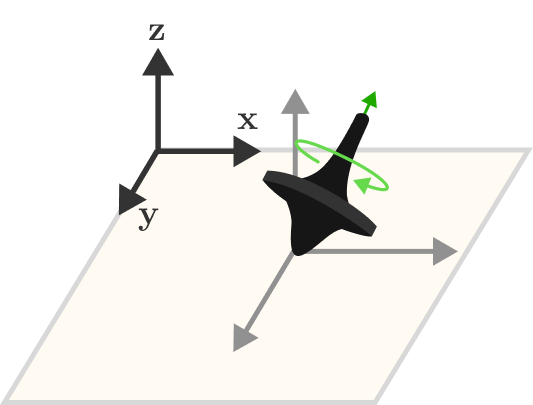In physics, an observable is a dynamic variable describing a state which can be measured. From a spinning top, we could observe the $x$-, $y$-, and $z$-components of the spin axis to find the spin vector. The angular velocity and kinetic energy would also be physical observables associated with the spin.

The easiest way to measure the direction of the spin vector might be to rotate your perspective. You could capture the orientation by taking a photo of the top from the three cardinal directions: one from above looking perpendicular to the $xy$-plane, and two others directed at the $xz$- and $yz$-planes. From these points of view, we can measure the $x-$, $y$-, and $z$-components of the spin vector and fully describe the orientation of the top.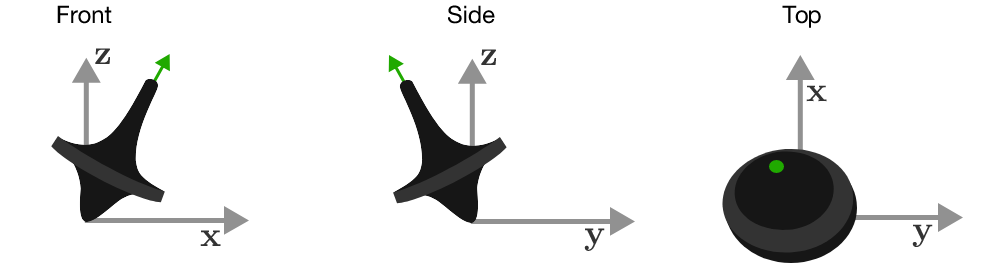We've had some success understanding neutrons as microscopic spinning tops, even though a spin vector that can only point up or down is an odd vector indeed. Let's see if we can measure the other neutron spin components with an SG analyzer by rotating our perspective.

Observables

So far, the particle spin axis seems to be acting much like a spatial vector along the $z$-direction, since it reverses direction when we flip our magnetic field aligned along that axis. The $\ket{\uparrow}$ and $\ket{\downarrow}$ states behave like spinning neutrons aligned along the positive and negative $z$-axes, respectively.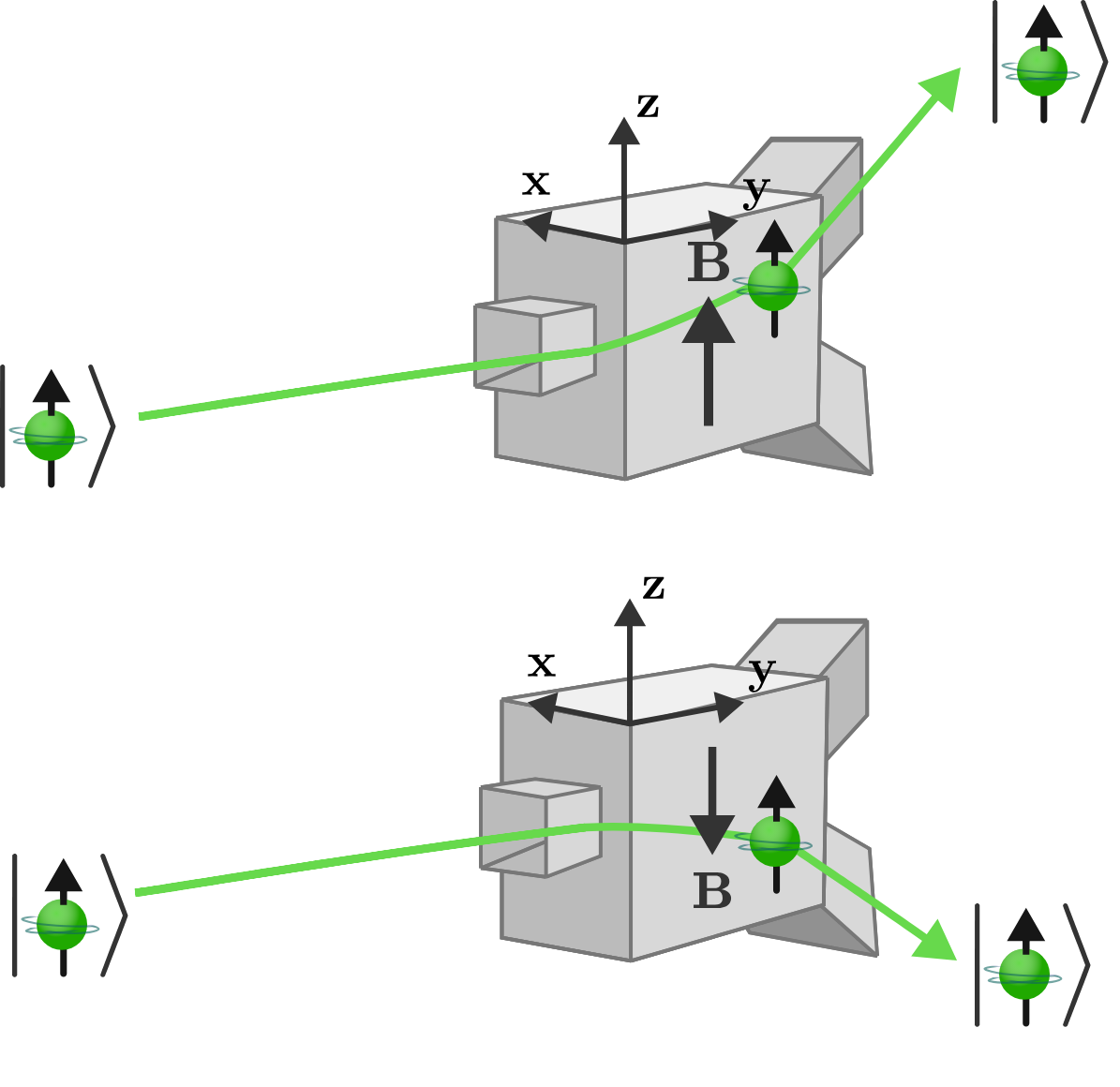To completely describe the spin of a top, we would need to observe its spin axis from several directions and record the $x$-, $y$-, and $z$-components.

How can we measure the $x$-component of the neutron spin vector using an SG analyzer?

Observables

Instead of flipping the second analyzer upside down, let’s rotate it by 90 degrees around the $y$-axis. Now the magnetic field is oriented along the positive $x$-axis, and we’ll refer to this device as an SG-$x$ analyzer.

Following the terminology we learned in the last quiz, the first SG-$z$ analyzer prepares the $\ket{\uparrow}$ and $\ket{\downarrow}$ states and filters them into two channels. Our physical model of these states suspects that they correspond to neutrons spinning around the positive and negative $z$-axes. The second SG-$x$ analyzer now measures the $x$-component of the $\mathbf{S}$ vector.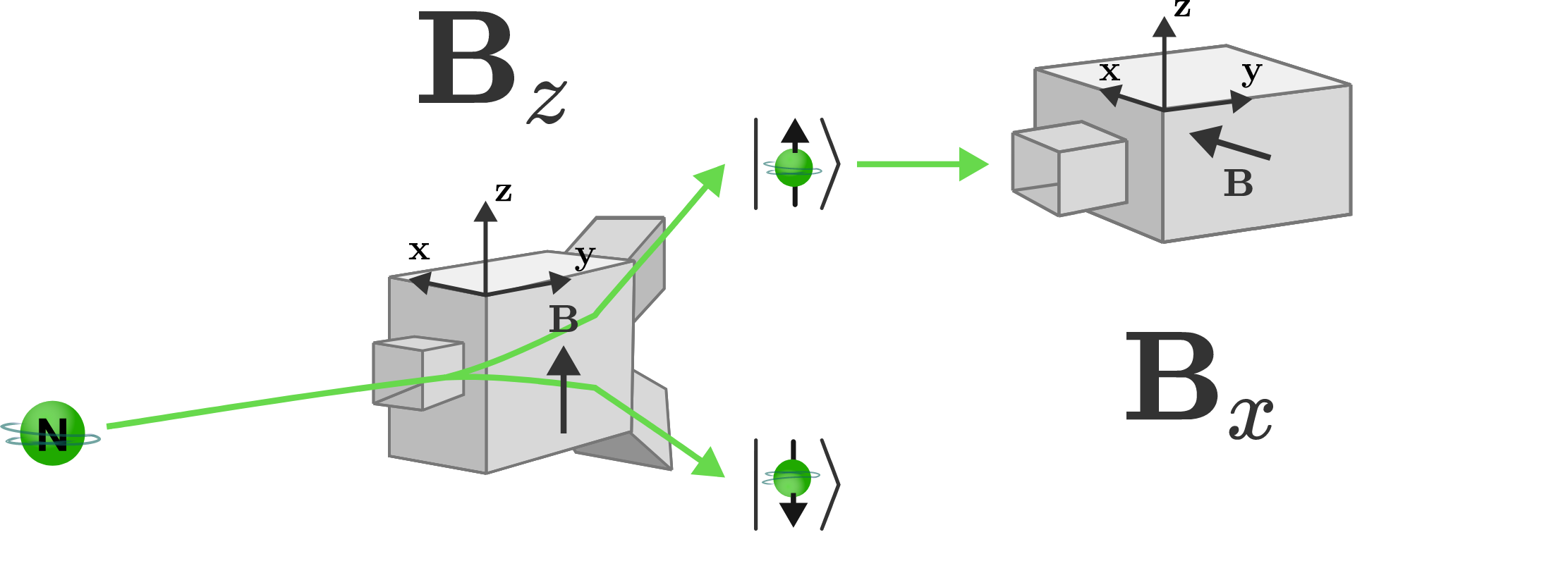If our understanding of the $\ket{\uparrow}$ and $\ket{\downarrow}$ states is correct, given a classical model of the magnetic field, what would we expect to observe from the second SG-$x$ analyzer?

Observables

The importance of this prediction calls for going through its derivation in detail:

When measuring the $z$-component of prepared $\ket{\uparrow}$ neutrons, we expect to see maximal “up” deflection, since the neutron spins are parallel with the magnetic field. Similarly, when we flipped the second SG analyzer upside-down, the deflection reversed since the spin magnetic moment was now anti-parallel to the magnetic field.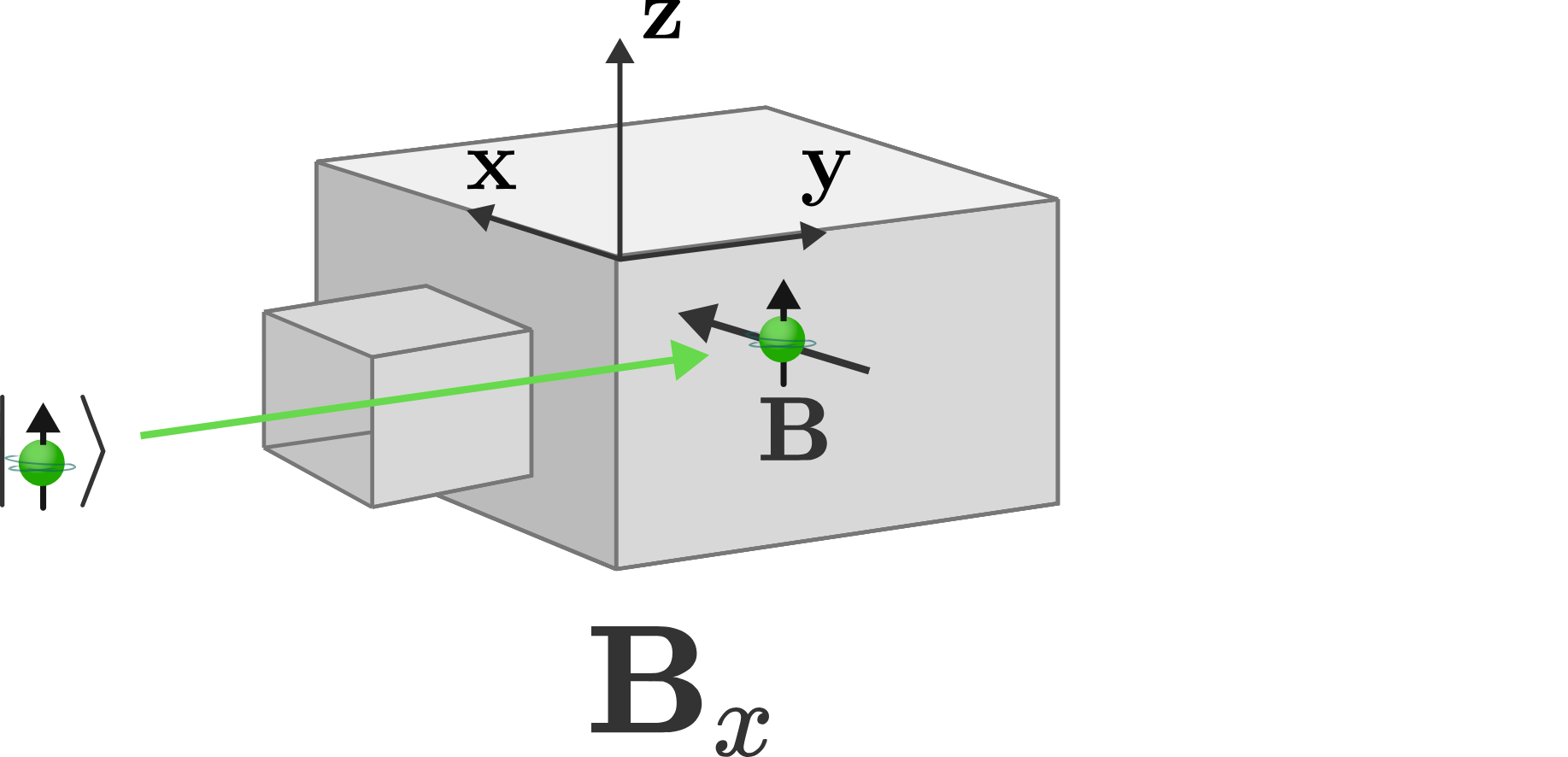Rotating the second SG analyzer by $\ang{90}$ makes the neutron spins aligned orthogonally to the magnetic field. Since the $x$-component of a vector in the $z$-direction is zero, we'd expect the deflection force to be zero. Thus, we would expect no neutrons to emerge from either $\ket{\uparrow}$ or $\ket{\downarrow}$ exit.

This is not what we observe: we again see a binary result, half of the neutrons are deflected as though their spin were aligned along the positive $x$-axis, and half as though they were aligned along the negative $x$-axis.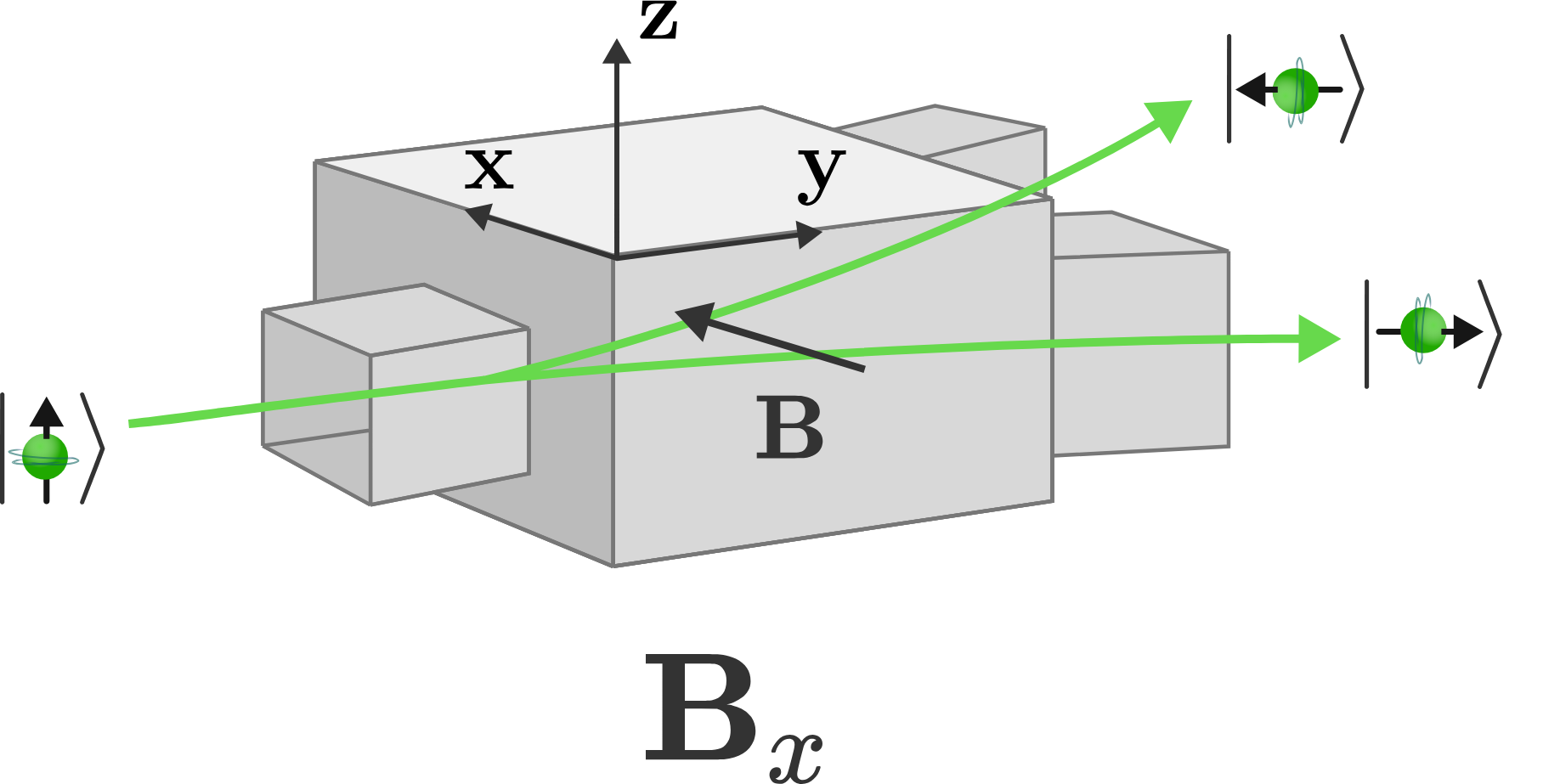Observables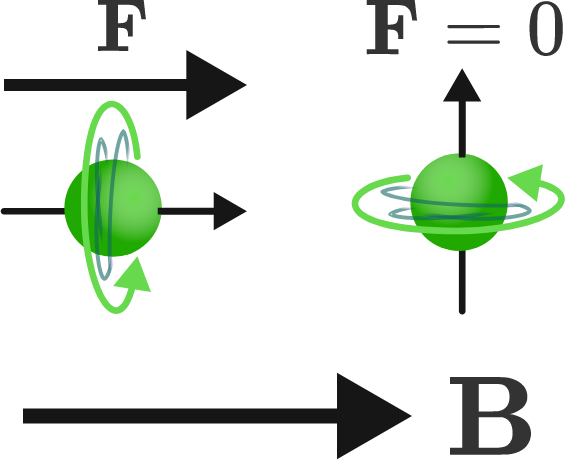The SG analyzer refuses to measure the expected $0$ deflection force even when measuring along an axis orthogonal to its spin. Instead, it stubbornly produces the same binary splitting as though half of the neutrons were aligned along each direction of the $x$-axis, which we will refer to as $\ket{\rightarrow}$ and $\ket{\leftarrow}.$

Somehow the spin vector $\mathbf{S}$ just got even stranger: particles observed to be aligned along the $z$-axis also seem to be simultaneously aligned along the $x$-axis.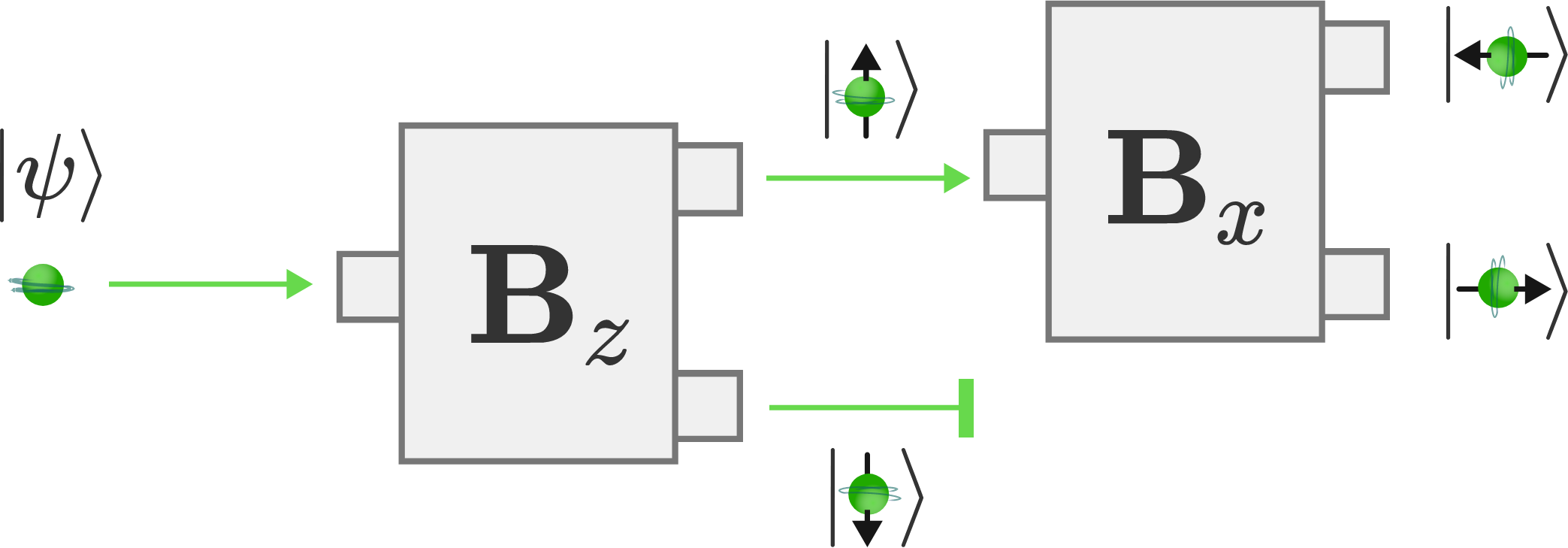A normal everyday vector cannot be aligned along two orthogonal axes simultaneously, but that appears to be exactly what we observe. In fact, we can repeat this measurement with other rotations of the second SG analyzer, and we continue to see the same binary result for any set or order of orthogonal measurements from which we'd expect $0$ deflection force.

What physical law of quantum objects might explain these observations?

Observables

Spin is a quantum property, meaning it can only be observed in a given set of quantized values, and in this case, $0$ is not one of those values, even though that would be the classically expected solution.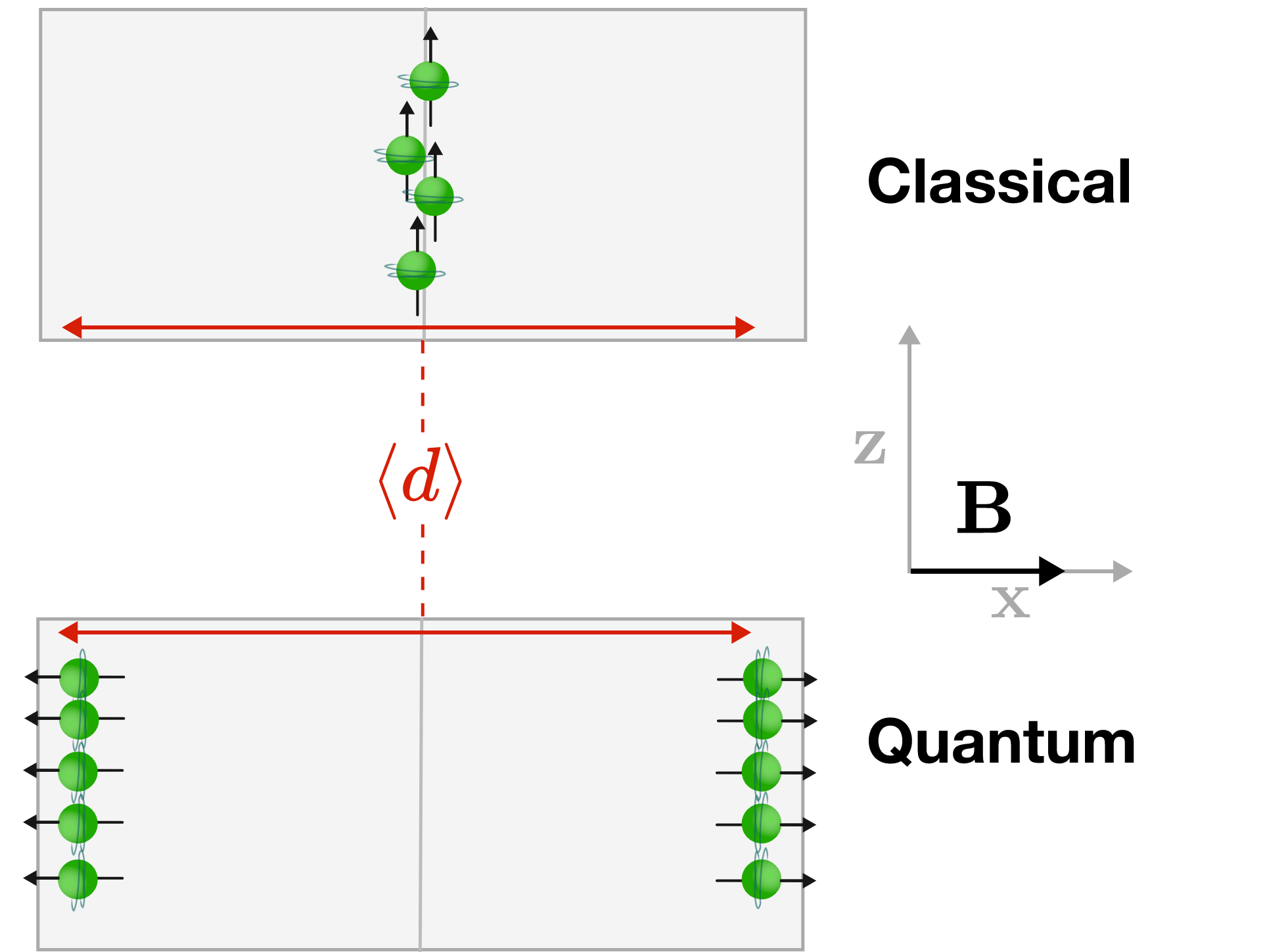But there is a glimmer of classical physics remaining: if we repeat this experiment many many times, the number of $\ket{\uparrow}$ neutrons which are measured to be $\ket{\rightarrow}$ and $\ket{\leftarrow}$ are equal--so the average deflection is zero, matching with the classical expectation:

At the limit of many quantum measurements, the average will always approach the classical result, even if individual experiments appear to violate the classical law. This is called the classical limit.

Let’s say we don’t rotate the second SG analyzer by $90^{\cdot}$ from the $z$-axis, but instead by an arbitrary angle, $\theta$. If we treat the deflection of the $\ket{\uparrow}$ and $\ket{\downarrow}$ states to be $+1$ and $-1,$ respectively, what would be the average deflection?

Hint: Calculate the classical limit of this experiment to find the average of many quantum measurements.

Observables

The SG analyzer will only ever observe one of the two allowed quantized spin values. Each corresponds to maximum deflection as though the neutrons were aligned parallel or antiparallel to the magnetic field. When measuring with an SG-$z$ analyzer, the only two observations are $\ket{\uparrow}$ and $\ket{\downarrow};$ when measuring with an SG-$x$ analyzer, the only two observations are $\ket{\leftarrow}$ and $\ket{\rightarrow}.$

Though these are the only two allowed observations, their distribution is biased to produce a statistical average matching the classical limit. For a rotation of $\theta$ away from the $z$-axis, this corresponds to an average deflection of $\cos{\theta}.$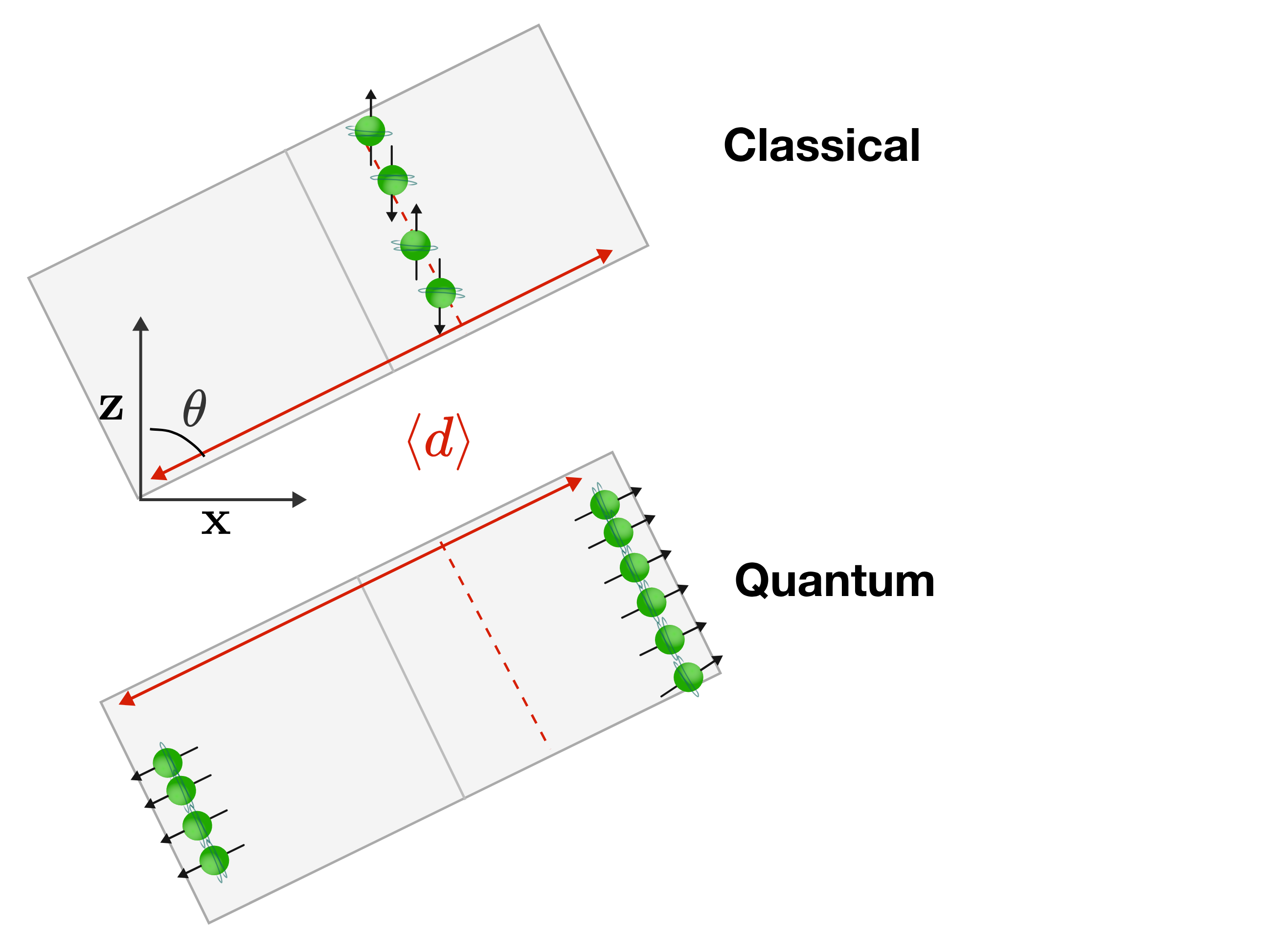The fact that the statistical average of a quantum measurement tends towards the classically expected result at the limit of many measurements explains in a large part why quantum behavior is so counterintuitive: Macroscopic objects consist of extraordinarily large numbers of microscopic particles, and we usually only observe their averages unless we're looking very closely.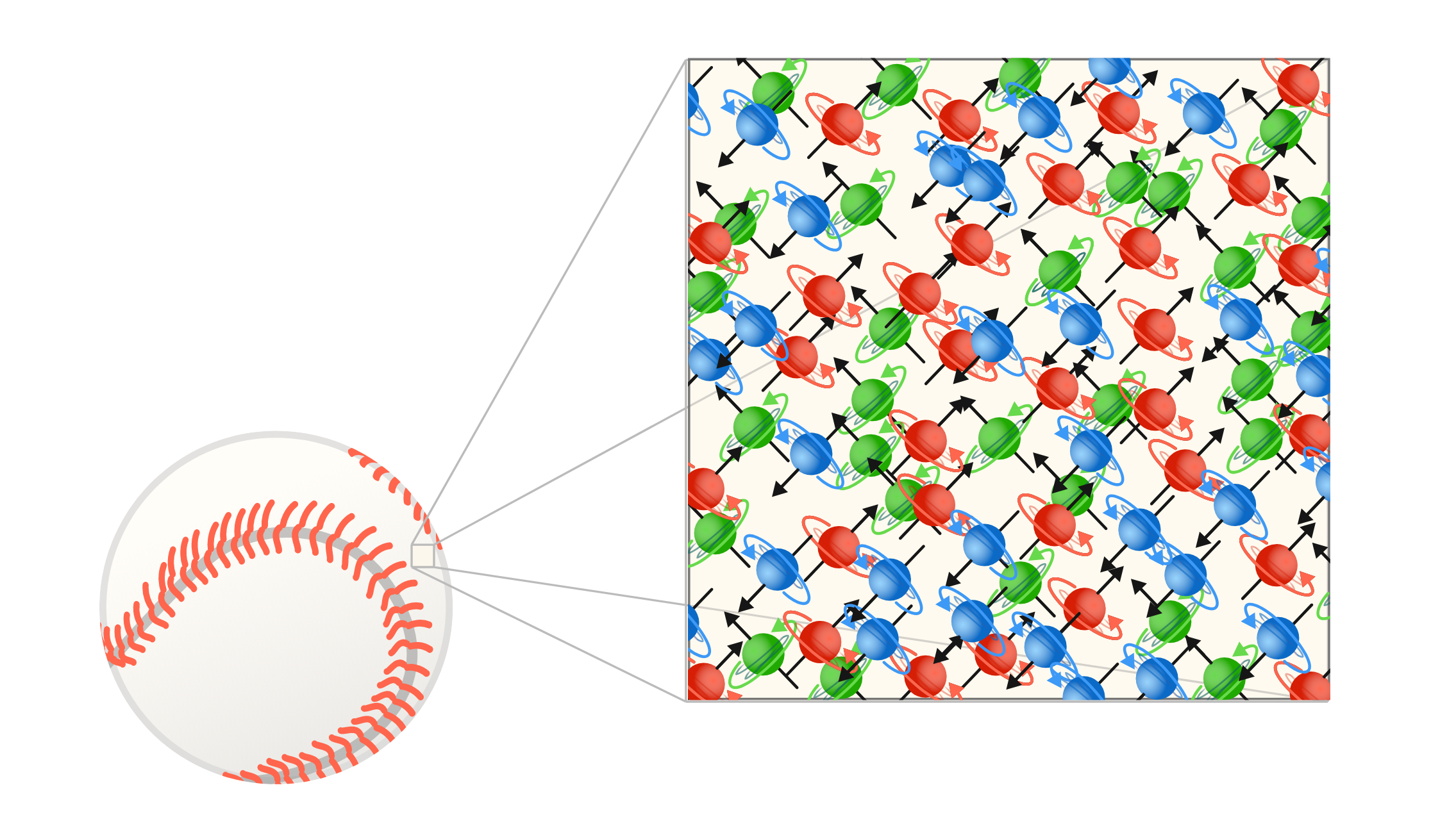ObservablesOur observations of neutron spin suggest it's similar to a spatial vector, insofar that it flips with the orientation of the analyzer. But in other ways, it seems to be fundamentally incompatible—it's hard to imagine a physical vector that can be aligned with two different directions in subsequent measurements.

As a sanity check, let’s add a third SG analyzer to the previous experiment, aligned like the first to measure the $z$-component of the neutron spin $\mathbf{S}$ again.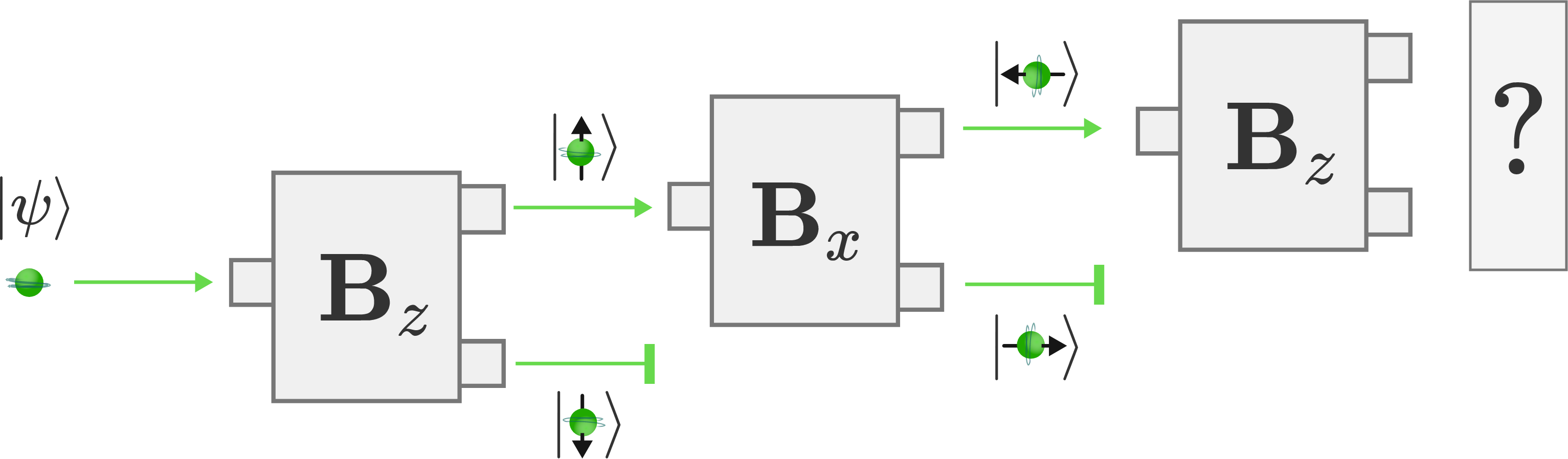Based on our results thus far, what channel(s) would you expect the neutrons to emerge from in the third analyzer?

Observables

We’ve already observed that the $\ket{\uparrow}$ state prepared by the SG-$z$ analyzer is robust to multiple successive measurements. Given this understanding, we’d expect that the third measurement would observe that all the neutrons have spin up along the $z$-axis, because that’s the state that we prepared at the first SG analyzer.

This is not what we observe. The neutrons leaving the third SG analyzer are split with a 50:50 probability into $\ket{\uparrow}$ and $\ket{\downarrow},$ even though we diverted all $\ket{\downarrow}$ neutrons away from the experiment with the first analyzer.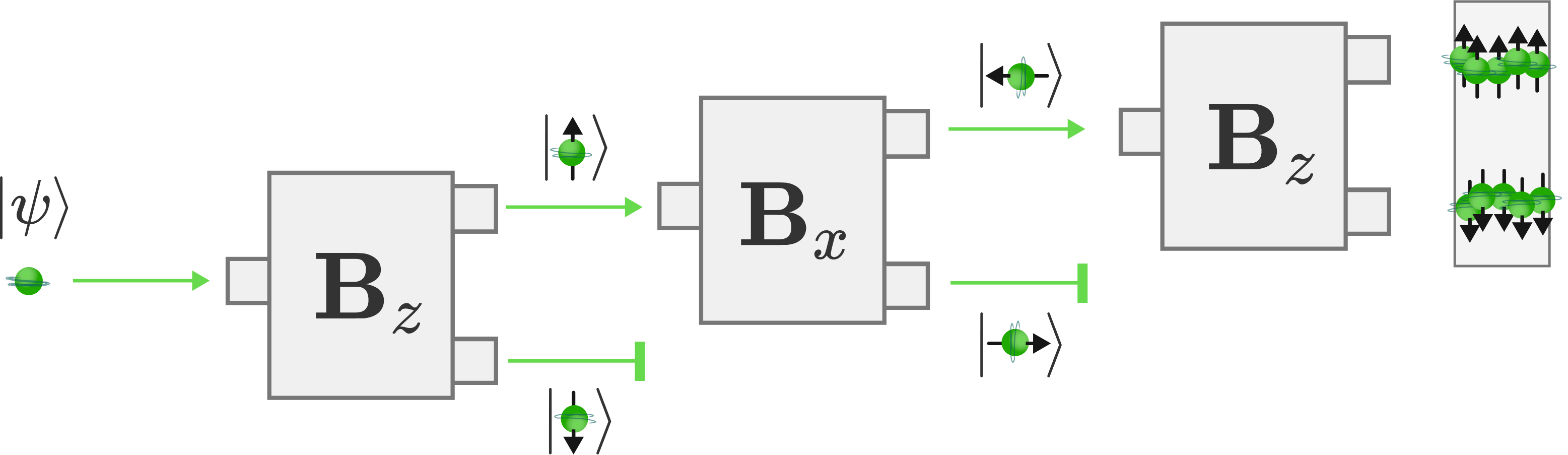How can we explain this contradictory result?

Observables

This shocking result demonstrates another key feature of quantum behavior:

Quantum measurements are not as gentle as classical measurements. Sometimes measuring a quantum state can change the state for all future measurements.

The unknown state $\ket{\psi}$ which emerged from our thermonuclear oven was never aligned along the positive and negative $z$-axes. By measuring the $z$-component of the spin, we changed the state into one of two possibilities: $\ket{\uparrow}$ and $\ket{\downarrow}$. Measuring another component caused the same result. The SG-$x$ analyzer reset the prepared $\ket{\uparrow}$ state into one of two completely different states: $\ket{\rightarrow}$ and $\ket{\leftarrow}.$

One might ask, "Can we be more clever in designing the experiment such that we don't disturb the system?" No, there is a fundamental incompatibility in trying to measure the spin component of a quantum object along two different directions. So we say that the $x$ and $z$ spin components are incompatible observables. We can’t ever know them both simultaneously.

Observables

Let’s revisit our classical model of spinning tops. Not being able to measure both the $\mathbf{S_z}$ and $\mathbf{S_x}$ spin components is completely different than the classical case where we can measure all three components of a spin vector easily. Only after determining all three of these components can we determine the direction that the spin vector is pointing.For quantum objects, the different spin components are incompatible. We can never know which way the neutrons are pointing. When we say “the spin is up”, we really mean only that the spin component along one axis is up. This property isn't permanent, since measuring the spin along any other axis will reset the spin and split it back into parallel and antiparallel states.

Observables

×